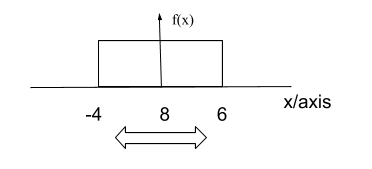# The Uniform Distribution

Contents

## Summary

• In uniform distribution the random variable is a continuous random variable
• The probability density function is calculated as:$f\left( x \right) \quad =\quad \frac { 1 }{ b\quad -\quad a }$
• Mean$=\quad \mu \quad =\quad \frac { A\quad +\quad B }{ 2 }$
• Variance$=\quad { \sigma }^{ 2 }\quad =\quad \frac { { (b\quad -\quad a) }^{ 2 } }{ 12 }$
• The cumulative distribution function is calculated by integrating the probability density function f(x) to give$\frac { x\quad -\quad a }{ b\quad -\quad a }$
• Standard deviation is the under root of variance$\sqrt { \frac { { (b\quad -\quad a) }^{ 2 } }{ 12 } }$

In uniform distribution you should know that random variable is a continuous random variable. In continuous uniform distribution it takes infinite number of real values in an interval.

For example, if we say that it is observed in a school, over a period of 2 months that the teacher arrives in school earliest by 4 minutes, before school starts or latest by 6 minutes after school starts.

(note: we are assuming that school starts at sharp 8 in the morning)

Thus looking at the figure below, we can deduce that the probability of a teacher entering class anywhere between 7:56 to 8:06 is constant.However, in order to find probability density function, we can say that, we know that the since, -4 till 6 are the only possibilities, we can thus say that the probability is greater than 0 only if the interval of x is between -4 and 6$\left[ -4\quad \le \quad x\quad \le \quad 6 \right]$.

Otherwise we can say that it is equal to 0 ( meaning, the teacher has taken a leave).We can thus write the uniform distribution as:

X ~ U(A, B)

Which in this case will be:

X ~ U(-4, 6)

Now, in order to find the interval between A and B we use the probability density function. Which is calculated as:$f\left( x \right) \quad =\quad \frac { 1 }{ b\quad -\quad a }$

In addition, if you are asked in a question, to find the mean we use the following formula:

Mean$=\quad \mu \quad =\quad \frac { A\quad +\quad B }{ 2 }$

But, we also need to understand how to derive this formula:$\int _{ -\infty }^{ +\infty }{ xf\left( x \right) dx } \quad =\quad \int _{ a }^{ b }{ x(\frac { 1 }{ b\quad -\quad a } ) } dx$$=\quad \frac { 1 }{ b\quad -\quad a } \int _{ a }^{ b }{ xdx }$$=\quad \frac { 1 }{ b\quad -\quad a } { (\frac { { x }^{ 2 } }{ 2 } ) }_{ a }^{ b }$$=\quad \frac { 1 }{ b\quad -\quad a } \left[ \frac { { b }^{ 2 }\quad -\quad { a }^{ 2 } }{ 2 } \right]$$=\quad \frac { 1 }{ b\quad -\quad a } \left[ \frac { (b\quad -\quad a)(b\quad +\quad a) }{ 2 } \right]$$=\quad \frac { b\quad +\quad a }{ 2 }$

Variance$=\quad { \sigma }^{ 2 }\quad =\quad \frac { { (b\quad -\quad a) }^{ 2 } }{ 12 }$

#### Cumulative Distribution Function

The cumulative distribution function of a continuous random variable, is known give the probabilities$P(X\quad \le \quad x)$ and is calculated by integrating the probability density function f(x) between the limits$-\infty$ and x.$\int _{ -\infty }^{ x }{ xf\left( x \right) dx } \quad =\quad \int _{ a }^{ x }{ x(\frac { 1 }{ b\quad -\quad a } ) } dx$$=\quad _{ a }^{ x }{ x }(\frac { 1 }{ b\quad -\quad a } )$$=\quad \frac { x\quad -\quad a }{ b\quad -\quad a }$

#### Example #1

Q. A random variable is uniformly distributed over the interval 2 to 10. Find its variance.

Solution:

We have been given the interval 2 to 10 so we know, a = 2 and b = 10

The formula for variance is$\frac { { (b\quad -\quad a) }^{ 2 } }{ 12 }$.

Plug, in the values to calculate the answer:$\frac { { (10\quad -\quad 2) }^{ 2 } }{ 12 } \quad =\quad 5.33$     Ans

#### Example #2

Q. A zero mean random signal is uniformly distributed between limits -a and +a and its mean square value is equal to its variance. Then the standard deviation of the signal is?

Solution:

We know that standard deviation is the under root of variance thus:$\sqrt { \frac { { (b\quad -\quad a) }^{ 2 } }{ 12 } }$

Next, the values of a and b are given to us:

a= -a and b = +a

Now plug the values into the formula above to get$\sqrt { \frac { { (a\quad -\quad (-a)) }^{ 2 } }{ 12 } \quad =\quad \frac { { (a\quad +\quad a) }^{ 2 } }{ 12 } \quad =\quad \frac { { 4a }^{ 2 } }{ 12 } } =\quad \frac { a }{ \sqrt { 3 } }$   Ans

### Example#3

Q. Suppose a train is delayed by approximately 60 minutes. What is the probability that train will reach by 57 minutes to 60 minutes?

Solution:

Interval of probability distribution a = 0 minutes b = 60 minutes

We need to find the probability density function with the given values of a and b between the probability$(57\quad \le \quad x\quad \le \quad 60)$:$f\left( x \right) \quad =\quad \frac { 3 }{ b\quad -\quad a } \quad =\quad \frac { 3 }{ 60\quad -\quad 0 } \quad =\quad \frac { 3 }{ 60 }$

We can thus, say the probability that train will reach by 57 minutes to 60 minutes is .